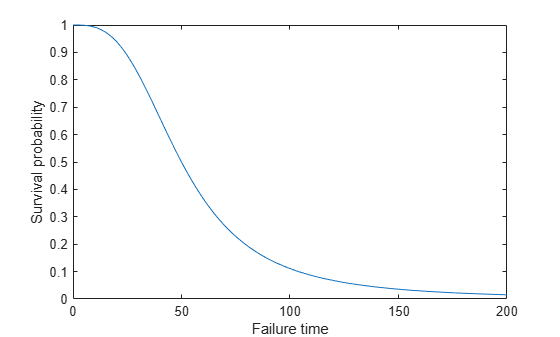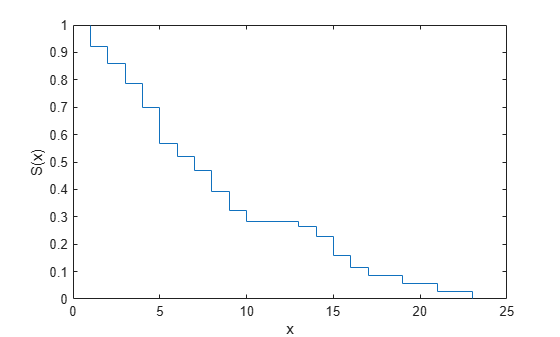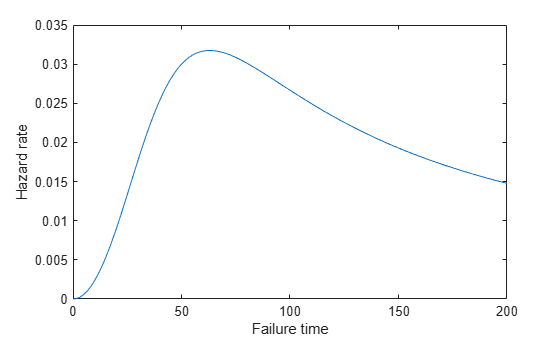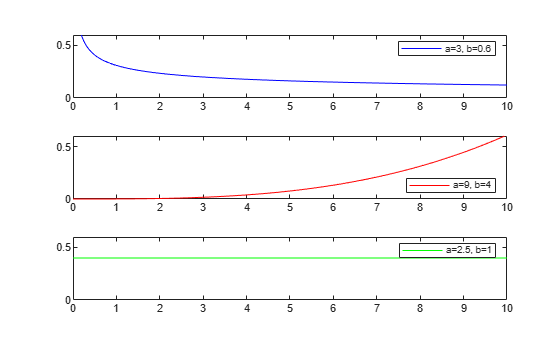## What Is Survival Analysis?

### Introduction

Survival analysis is time-to-event analysis, that is, when the outcome of interest is the time until an event occurs. Examples of time-to-events are the time until infection, reoccurrence of a disease, or recovery in health sciences, duration of unemployment in economics, time until the failure of a machine part or lifetime of light bulbs in engineering, and so on. Survival analysis is a part of reliability studies in engineering. In this case, it is usually used to study the lifetime of industrial components. In reliability analyses, survival times are usually called failure times as the variable of interest is how much time a component functions properly before it fails.

Survival analysis consists of parametric, semiparametric, and nonparametric methods. You can use these to estimate the most commonly used measures in survival studies, survivor and hazard functions, compare them for different groups, and assess the relationship of predictor variables to survival time. Some statistical probability distributions describe survival times well. Commonly used distributions are exponential, Weibull, lognormal, Burr, and Birnbaum-Saunders distributions. Statistics and Machine Learning Toolbox™ functions `ecdf` and `ksdensity` compute the empirical and kernel density estimates of the cdf, cumulative hazard, and survivor functions. `coxphfit` fits the Cox proportional hazards model to the data.

### Censoring

One important concept in survival analysis is censoring. The survival times of some individuals might not be fully observed due to different reasons. In life sciences, this might happen when the survival study (e.g., the clinical trial) stops before the full survival times of all individuals can be observed, or a person drops out of a study, or for long-term studies, when the patient is lost to follow up. In the industrial context, not all components might have failed before the end of the reliability study. In such cases, the individual survives beyond the time of the study, and the exact survival time is unknown. This is called right censoring.

During a survival study either the individual is observed to fail at time T, or the observation on that individual ceases at time c. Then the observation is min(T,c) and an indicator variable Ic shows if the individual is censored or not. The calculations for hazard and survivor functions must be adjusted to account for censoring. Statistics and Machine Learning Toolbox functions such as `ecdf`, `ksdensity`, `coxphfit`, and `mle` account for censoring.

### Data

Survival data usually consists of the time until an event of interest occurs and the censoring information for each individual or component. The following table shows the fictitious unemployment time of individuals in a 6-month study. Two individuals are right censored (indicated by a censoring value of 1). One individual was still unemployed after the 24th week, when the study ended. Contact with the other censored individual was lost at the end of the 21st week.

Unemployment Time (Weeks)Censoring
140
230
70
211
190
160
241
80

Survival data might also include the number of failures at a certain time (the number of times a particular survival or failure time was observed). The following table shows the simulated time until a light-emitting diodes drops to 70% of its full light output level, in hours, in an accelerated life test.

Failure Time (hrs)Frequency
86006
1530019
2200011
2860020
3530017
4200014
487008
554002
621000
688002

Data might also have information on the predictor variables, to use in semi-parametric regression-like methods such as Cox proportional hazards regression.

Time Until Recovery (weeks)CensoringGenderSystolic Blood PressureDiastolic Blood Pressure
121Male12493
200Female10977
70Female12583
130Male 11775
91Male12280
150Female12170
171Male13088
80Female11582
140Male11886

### Survivor Function

The survivor function is the probability of survival as a function of time. It is also called the survival function. It gives the probability that the survival time of an individual exceeds a certain value. Since the cumulative distribution function, F(t), is the probability that the survival time is less than or equal to a given point in time, the survival function for a continuous distribution, S(t), is the complement of the cumulative distribution function:

S(t) = 1 – F(t).

The survivor function is also related to the hazard function. If the data has the hazard function, h(t), then the survivor function is

`$S\left(t\right)=\mathrm{exp}\left(-\underset{0}{\overset{t}{\int }}h\left(u\right)du\right),$`

which corresponds to

`$S\left(t\right)=\mathrm{exp}\left(-H\left(t\right)\right),$`

where H(t) is the cumulative hazard function.

#### Burr Distribution Survivor Function

Calculate and plot the survivor function of a Burr distribution with parameters `50`, `3`, and `1`.

```x = 0:0.1:200; figure() plot(x,1-cdf('Burr',x,50,3,1)) xlabel('Failure time'); ylabel('Survival probability');```#### Survivor Function from Data

This example shows how to estimate the survivor function from data.

`load readmissiontimes`

The column vector `ReadmissionTime` shows the readmission times for 100 patients. The column vector `Censored` has the censorship information for each patient, where 1 indicates censored data, and 0 that indicates the exact readmission times are observed. This data is simulated.

`[ReadmissionTime Censored]`
```ans = 100×2 5 1 3 1 19 0 17 0 9 0 16 0 4 0 2 0 3 0 15 0 ⋮ ```

The first two readmission times, `5` and `3`, are both censored.

Display the empirical survivor function with censoring using `ecdf` with the name-value pair arguments `'function','survivor'` and `'censoring',Censored`.

`ecdf(ReadmissionTime,'censoring',Censored,'function','survivor')`### Hazard Function

The hazard function gives the instantaneous failure rate of an individual conditioned on the fact that the individual survived until a given time. That is,

`$h\left(t\right)=\underset{\Delta t\to 0}{\mathrm{lim}}\frac{P\left(t\le T`

where Δt is a very small time interval. The hazard rate, therefore, is sometimes called the conditional failure rate. The hazard function always takes a positive value. However, these values do not correspond to probabilities and might be greater than 1.

The hazard function is related to the probability density function, f(t), cumulative distribution function, F(t), and survivor function, S(t), as follows:

`$h\left(t\right)=\frac{f\left(t\right)}{S\left(t\right)}=\frac{f\left(t\right)}{1-F\left(t\right)},$`

which is also equivalent to

`$h\left(t\right)=-\frac{d}{dt}\mathrm{ln}S\left(t\right).$`

So, if you know the shape of the survival function, you can also derive the corresponding hazard function.

#### Burr Distribution Hazard Function

Calculate and plot the hazard function of a Burr distribution with parameters `50`, `3`, and `1`.

```x = 0:1:200; Burrhazard = pdf('Burr',x,50,3,1)./(1-cdf('Burr',x,50,3,1)); figure() plot(x,Burrhazard) xlabel('Failure time'); ylabel('Hazard rate');```#### Weibull Hazard Functions

There are different types of hazard functions. The previous figure shows a situation when the hazard rate increases for the early time periods and then gradually decreases. The hazard rate might also be monotonically decreasing, increasing, or constant over time. The following figure shows examples of different types of hazard functions for data coming from different Weibull distributions.

```figure ax1 = subplot(3,1,1); x1 = 0:0.05:10; hazard1 = pdf('wbl',x1,3,0.6)./(1-cdf('wbl',x1,3,0.6)); plot(x1,hazard1,'color','b') set(ax1,'Ylim',[0 0.6]); legend(ax1,'a=3, b=0.6'); ax2 = subplot(3,1,2); x2 = 0:0.05:10; hazard2 = pdf('wbl',x2,9,4)./(1-cdf('wbl',x2,9,4)); plot(x2,hazard2,'color','r') set(ax2,'Ylim',[0 0.6]); legend(ax2,'a=9, b=4','location','southeast'); ax3 = subplot(3,1,3); x3 = 0:0.05:10; hazard3 = pdf('wbl',x3,2.5,1)./(1-cdf('wbl',x3,2.5,1)); plot(x3,hazard3,'color','g') set(ax3,'Ylim',[0 0.6]); legend(ax3,'a=2.5, b=1');```In the third case, the Weibull distribution has a shape parameter value of `1`, which corresponds to the exponential distribution. The exponential distribution always has a constant hazard rate over time.

 Cox, D. R., and D. Oakes. Analysis of Survival Data. London: Chapman & Hall, 1984.

 Lawless, J. F. Statistical Models and Methods for Lifetime Data. Hoboken, NJ: Wiley-Interscience, 2002.

 Kleinbaum, D. G., and M. Klein. Survival Analysis. Statistics for Biology and Health. 2nd edition. Springer, 2005.